# Symmetry - Definition with Examples

The Complete K-5 Math Learning Program Built for Your Child

• 40 Million Kids

Loved by kids and parent worldwide

• 50,000 Schools

Trusted by teachers across schools

• Comprehensive Curriculum

Aligned to Common Core

## What is Symmetry?

Look at these two images of butterflies. What difference do you see?The first butterfly looks exactly the same from both the left and right sides while the second butterfly does not look the same from the left and right sides.

The images which can be divided into identical halves are called symmetrical. The images that cannot be divided into identical halves are asymmetrical.

You must have seen the following symmetrical objects in your surroundings:## Line of symmetry

Any line splitting a shape into two parts such that the two parts are the same is called a line of symmetry. These parts are also said to be symmetrical to each other.

For instance, the image below shows a line of symmetry which splits the red outlined shape into two parts that are exactly the same.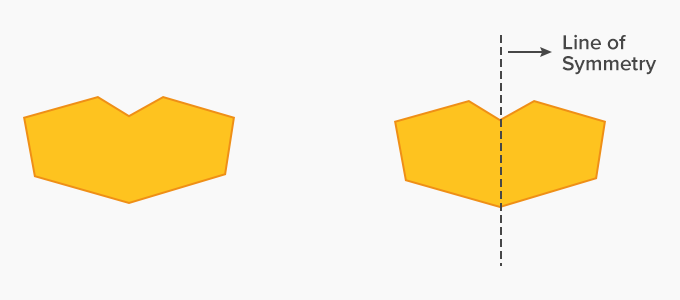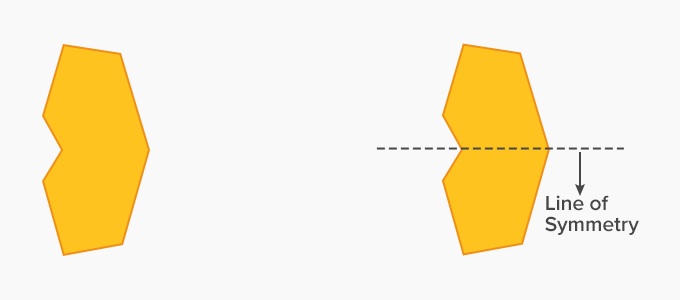Based on the above examples, we obtain the following observations:

• The sides of the image split up by the line of symmetry, must look the same[c].

• If we fold the paper (on which image is drawn) along the line of symmetry, each part of the image will completely overlap the other part.

The above observations will help us find the line of symmetry in any shape.

Example 1: Which of the following shapes does not have a line of symmetry?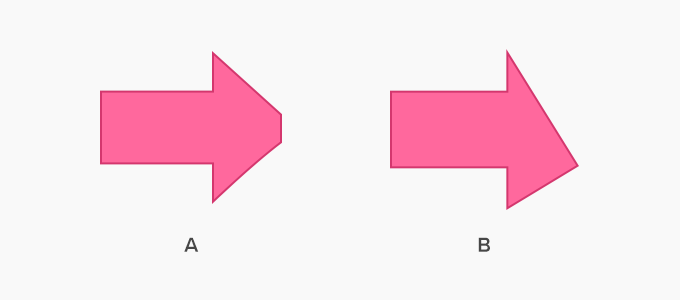Solution

If we fold both the papers from top to down as shown in A1 and B1, we get a line of symmetry in A but not in B. If we fold both the papers from left to right as shown in A2 and B2, we get no line of symmetries in both A and B.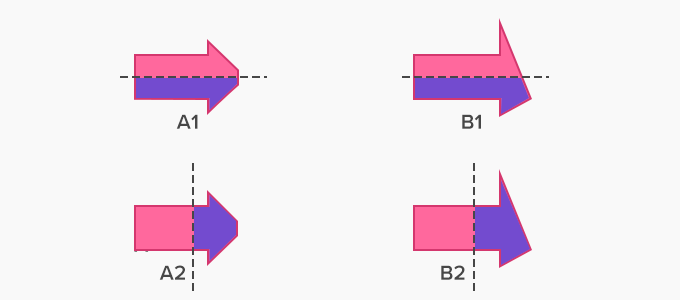## Shapes with more than one lines of symmetry

Can we have more than one line of symmetry? The answer is yes. The table below shows some examples of shapes/figures with more than one line of symmetry.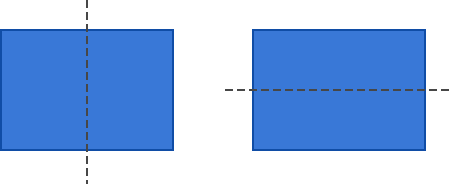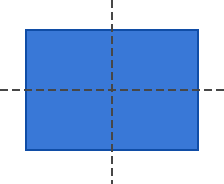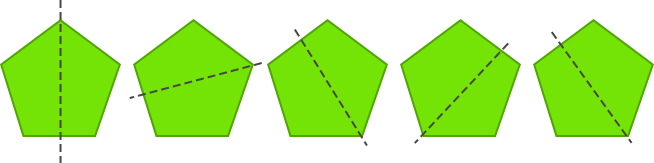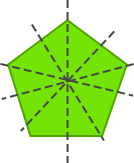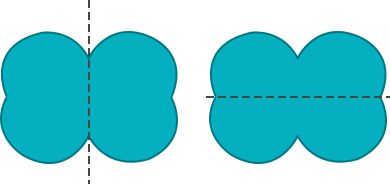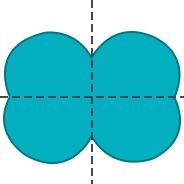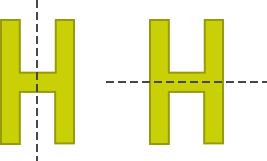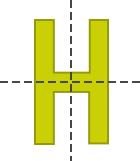Real-life examples of symmetry

• Reflection of trees in clear water and reflection of mountains in a lake.

• Wings of most butterflies are identical on the left and right sides.

• Some human faces are the same on the left and right side.

• People can also have a symmetrical mustache.Example 3: Which of the following is not a line of symmetry?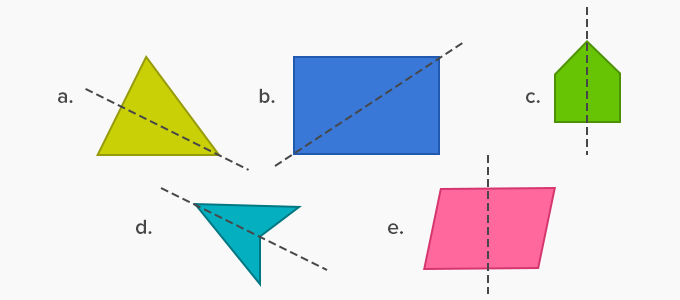Answer:  (b) and (e) does not have a line of symmetry.

Example 4: Given below is a left part of a picture and its line of symmetry. Complete the picture.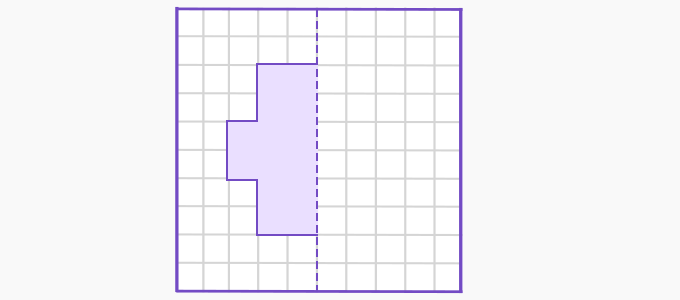Solution:

The complete picture is as shown below.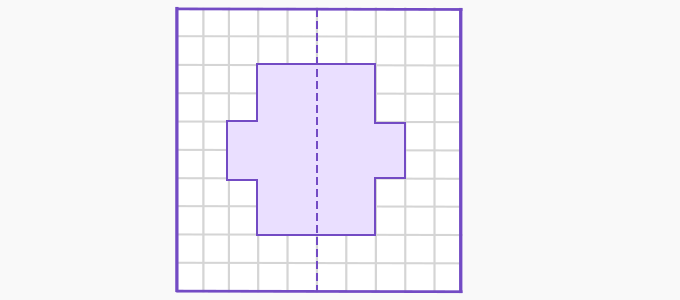The other half should be exactly the same as the given half. We can use the grids to find the other half. We look at each vertex of the yellow part and measure its distance from the line of symmetry. Now we draw each vertex corresponding to the yellow part vertices on the purple section, keeping the distance from the line of symmetry.

Won Numerous Awards & Honors